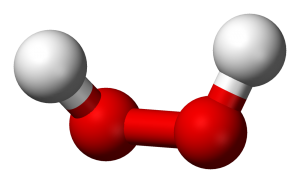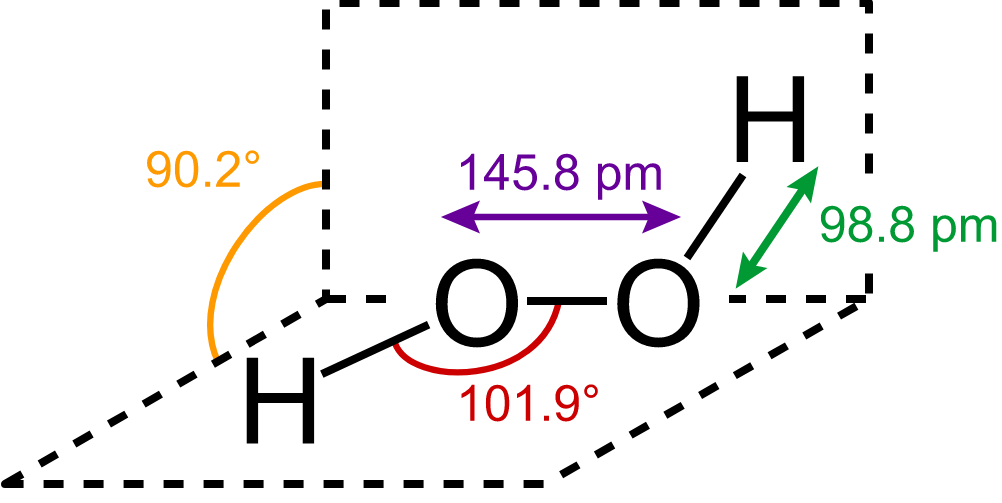# Hydrogen Peroxide - Structure, Preparation, Properties & Uses

## What is Hydrogen Peroxide?

Hydrogen peroxide is the simplest kind of peroxide available (oxygen-oxygen single bond). It is a colorless liquid and is used in aqueous solution for safety reasons. Its pure form will cause severe burns if heated to boiling temperature. It acts as a bleaching agent and is also used as a disinfectant. Concentrated hydrogen peroxide is a very reactive oxygen species and is used as a propellant in rocketry. The chemical formula for hydrogen peroxide is H2O2, it is often referred to as water with one more oxygen atom.Hydrogen Peroxide 3D ball structural representation

### Structure of Hydrogen Peroxide:

The structure of hydrogen peroxide is non-planar. This structure is also known as open book structure. The following diagram will clearly show what an open book structure means:Hydrogen Peroxide Structure

There are two planes in this structure and each plane has one O-H pair, the angle between both the planes is 90.2°, The O-O bond length is 145.8 pm and the O-H bond length is 98.8 pm(which is equal to 9.88 × 10-13 m).

### Preparation of Hydrogen Peroxide:

Let us have a look at the various methods of preparation for hydrogen peroxide:

1. When barium peroxide is acidified and the excess water is removed by the process of evaporation under reduced pressure, we obtain hydrogen peroxide. The following reaction will clarify this:

$\large \frac{P}{\rho }+g\;z+\frac{v^{2}}{2}=k$

$\large \frac{P}{\rho }+g\;z+\frac{v^{2}}{2}=k$

$BaO_2.8H_2O(s) + H_2SO_4(aq) \rightarrow BaSO_4(s) + H_2O_2 (aq) + 8H_2O(l)$

1. When acidified sulphate solution is electrolyzed at high current density, peroxodisulphate is obtained. Peroxodisulphate is then hydrolyzed to get hydrogen peroxide.
$2HSO_4^- (aq)~ \underrightarrow{Electrolysis} ~HO_3SOOSO_3H (aq) ~\underrightarrow{Hydrolysis} ~ 2HSO_4^- (aq) + 2H^+ (aq) + H_2O_2(aq)$

### Properties of Hydrogen Peroxide:

#### Physical properties:

• In pure state hydrogen peroxide is almost colorless (very pale blue) liquid.
• It melts at 272.4 K and has a boiling point of 423 K (extrapolated).
• It is miscible in water in all proportions and forms hydrates.

#### Chemical properties:

Hydrogen peroxide in both acidic and basic medium acts as an oxidizing as well as the reducing agent. The following reactions will give a clear picture:

• Oxidizing nature in an acidic medium
$PbS (s) + 4H_2O_2 (aq) \rightarrow PbSO_4(s) + 4H_2O(l)$
• The reducing nature in an acidic medium
$HOCl + H_2O_2 \rightarrow H_3O^+ + Cl^- + O_2$
• Oxidizing nature in a basic medium
$Mn^{2+} + H_2O_2 \rightarrow Mn^{4+} + 2OH^-$
• Reducing nature in a basic medium
$I_2 + H_2O_2 + OH^- \rightarrow 2I^- + 2H_2O + O_2$

### Storage of Hydrogen Peroxide:

Hydrogen peroxide decomposes when exposed to sunlight, this process is catalyzed by traces of alkali metals. Therefore, hydrogen peroxide is stored in wax-lined glass or plastic containers and kept in dark. It should also be kept away from dust particles because dust can induce explosive decomposition of this compound.

### Uses of Hydrogen Peroxide:

Since hydrogen peroxide acts as both an oxidizing as well as a reducing agent and it has a vast area of application:

1. The textile and paper industry use it as a bleaching agent.
2. It is used as hair bleach in our day to day life and also as a mild disinfectant.
3. It is used for the synthesis of tartaric acid, food products, and many pharmaceuticals.
4. It is used in the manufacture of chemicals which in turn are used in making of high-quality detergents.
5. Its most significant use is in environmental chemistry where it is used in pollution control treatment of domestic waste and industrial effluents.

The topics are discussed in brief, for any further query install Byju’s, the learning app or watch the videos on Byju’s YouTube channel.

#### Practise This Question

Hydrogen peroxide is reduced by